# Monolayer Ti2C MXene: manipulating magnetic properties and electronic structures by an electric field†

Peng Lv , Yan-Li Li* and Jia-Fu WangDepartment of Physics, School of Science, Wuhan University of Technology, Wuhan, Hubei 430070, China. E-mail: liyanli128@whut.edu.cn

Received 30th January 2020 , Accepted 24th March 2020

First published on 24th March 2020

Two-dimensional (2D) layered Ti2C MXene has been synthesized experimentally, and the magnetism of monolayer Ti2C MXene has been predicted theoretically. In this study, based on first-principles calculations, five magnetic configurations of monolayer Ti2C were constructed to predict the magnetic ground state. We have found that the antiferromagnetic (AFM) state has the lowest energy. By applying an external electric field, monolayer Ti2C changes from an AFM semiconductor to a ferrimagnetic (FIM) semiconductor, half-metal, magnetic metal, non-magnetic (NM) metal, and NM semiconductor. When the electric field increases beyond a certain value, the magnetic moments of Ti atoms sharply decrease. With the increase in the electric field, the effective masses decrease significantly, carrier mobility increases and conductivity increases. The magnetic anisotropy energies were calculated and the results showed that the out-of-plane direction was the magnetic easy axis. Using the mean-field approximation method, the Néel temperature of monolayer Ti2C is estimated to be 50 K. By applying an electric field, the Néel temperature significantly decreases, which shows that the electric field can effectively reduce the high Néel temperature. Therefore, our research suggests that the magnetic and electronic properties of 2D materials can be manipulated by an external electric field, which provides a feasible direction for the tuning of nanomagnetic devices.

## Introduction

2D materials have attracted extensive attention owing to their unique properties. In 2004, Geim and Novoselov et al. obtained single-layer graphene by mechanical exfoliation.1 Since then, more and more 2D materials have been discovered, such as transition metal dichalcogenides (TMDs),2 hexagonal boron nitride (h-BN),3 transition metal oxides (TMOs),4 phosphorene,5 black phosphorous,6 borophene7 and silicene.43 These 2D materials have unique optical, electronic, physical, chemical and mechanical properties that have led to worldwide research. However, these materials cannot be used in the field of spin as most of their magnetic properties are weak or even non-magnetic.

Recently, transition metal carbides and nitrides, i.e., MXenes, a new family of 2D materials, which are similar to graphene, have been synthesized from the MAX phases using hydrofluoric acid (HF) solutions and sonication.8,9 The chemical formula of the MAX phases is Mn+1AXn, where “M” represents an early transition metal, “A” is mainly an A group element from groups III–VI, “X” refers to carbon and/or nitrogen, and n = 1, 2 or 3. So far, more than 70 kinds of MAX phases have been synthesized experimentally, e.g., Ti2AlC, Ti2AlN, V2AlC, Nb2AlC, Ti3AlC2, Ti3SiC2, Zr3AlC2, Ti4AlN3,V4AlC3, Nb4AlC3, Ta4AlC3, etc.10 Because the M–X bonds in the MAX phases are stronger than the M–A bonds, the A-element layers can be etched away to produce 2D layered MXenes.8,9 So far, more than 20 types of MXenes have been synthesized, such as Ti2C,9 Ti3C2,8 Ti2N,11 Ti4N3,12 V2C,13 Nb2C,13 V4C3,14 Nb4C3,15 Ta4C3,9 Mo2C16 and Zr3C2.17

MXenes having applications in electronics, optics, energy storage, Li-ion batteries and biomaterials have been experimentally discovered.18,19 Many theoretical predictions suggest that MXenes have attractive prospects, such as magnetism,20 thermoelectricity,21 optics22 and communication.23 Most theoretical studies on MXenes are concentrated on electrochemical energy storage and conversion, such as batteries44 and supercapacitors.45 Only a few layers of Tin+1Xn were predicted to be magnetic mainly due to the 3d-orbital electron contributions of the surface Ti atoms.24 Compared with the unpassivated Ti2C, the O-passivated Ti2C doped with a B element not only reduces the stiffness but also extends the critical strain.25 The magnetic monolayer Ti2C has good application prospects in Li-ion batteries (LIBs).26 Therefore, the monolayer Ti2C MXene has gained extensive attention for its attractive and unique properties.

Magnetic anisotropy is an important property of magnetic crystals. In the different directions of magnetization, the magnetic anisotropy energy (MAE) of magnetic crystals is different. The MAE of magnetic crystals is the lowest in the magnetic easy direction and it is the highest in the magnetic difficult direction. The magnetic anisotropy transition between in-plane magnetic anisotropy and perpendicular magnetic anisotropy can be induced by applying strain.46 Uniaxial strain,47 different stacking patterns48 and electric field20 can affect the MAE.

Recent studies have shown that applying an external electric field to 2D materials can regulate the properties, including the magnetic state, magnetic order, magnetic moments, Curie temperature (TC), Néel temperature (TN), magnetization direction, magnetic anisotropy, band gap, conductivity, electron transport, optics, as well as electronic phase transitions.20,27,28,49 Actually, applying an external electric field to 2D materials can regulate the work function, one of the important parameters for low contact barrier electrodes and various field-emitting devices.50

Based on these studies above, we conducted research on the magnetic properties and electronic structures of the monolayer Ti2C by applying an external vertical electric field (E).

## Computational methods

In this work, the first-principles calculations were performed within the framework of density functional theory (DFT) by using the Vienna Ab initio Simulator Package (VASP).29 The exchange–correlation function adopts the Perdew–Burke–Ernzerhof (PBE) of the generalized gradient approximation (GGA).30 For the geometry optimization, the plane-wave cutoff energy was set as 520 eV in the projector augmented wave (PAW) potential.31 The supercell of 2 × 2 × 1 was used to calculate the different magnetic configurations of monolayer Ti2C and the Monkhorst–Pack k-mesh was 24 × 24 × 1 in the Brillouin zone. To ensure optimization and self-consistent convergence, the energy and force criteria were set to be 10−6 eV and 10−3 eV Å−1, respectively. In addition, there were few changes in the electronic structures when the spin–orbit coupling (SOC) effect was taken into consideration. So, the SOC effect was not taken into consideration in the electronic structures. However, the SOC effect was considered when calculating the MAE. For the calculation of the MAE, the cutoff energy and energy convergence criteria of 600 eV and 10−8 eV were applied, respectively. In order to avoid the interactions between layers, the vacuum space of monolayer Ti2C in the direction of c was 20 Å.

The structure of monolayer Ti2C is shown in Fig. 1. As shown in Fig. 1(a), from the top view, Ti and C atoms form a hexagon. As shown in Fig. 1(b), from the side view, the top Ti atoms (named Ti1), middle C atoms and bottom Ti atoms (named Ti2) form a “sandwich” structure. An external E was applied in the positive direction of the c axis. In order to confirm the ground state magnetic configuration of the monolayer Ti2C, we compared the total energies of the NM, ferromagnetic (FM) and AFM states. Fig. 1(c–f) show the spin polarization directions of the FM state and AFM state. For the AFM configurations, we set up three types:25,32 A-AFM, C-AFM and G-AFM states. (1) A-AFM state in Fig. 1(d): intralayer Ti atoms constitute the FM state while interlayer Ti atoms form the AFM state. (2) C-AFM state in Fig. 1(e): intralayer Ti atoms constitute the AFM state while interlayer Ti atoms form the FM state. (3) G-AFM state in Fig. 1(f): both intralayer and interlayer Ti atoms constitute the AFM states. Generally, the PBE method underestimates the interactions of 3d-orbital electrons, so the PBE+U (where U = 4 eV)20,25 method was also employed to compare the energies of the five magnetic configurations.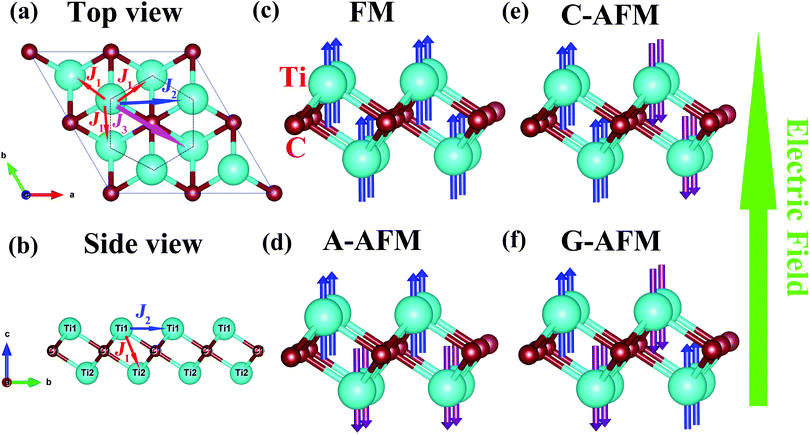Fig. 1 The structure of monolayer Ti2C: (a) top view and (b) side view. The magnetic configurations of (c) FM, (d) A-AFM, (e) C-AFM and (f) G-AFM states, respectively. The arrows on the Ti atoms are the directions of spin. The green arrow on the right shows the direction of the electric field.

## Results and discussion

The results of PBE and PBE+U methods are shown in Table 1. The results of the five magnetic configurations are in good agreement with the available results.25,26,32–35 The results of C-AFM and G-AFM states are almost the same. The 3d-orbital electrons of Ti (3d34s1) atom are unsaturated states. As shown in Table 1, the magnetic moments of Ti atoms are mainly contributed to by 3d-orbital electrons. The total magnetic moment of the FM state is 1.914 μB per unit cell, which is consistent with the available results: 1.92 μB,24 1.85 μB36 and 1.91 μB.37 The total magnetic moment of the FM state is mainly contributed to by Ti atoms, in which the magnetic moments of each Ti atom and C atom are 0.676 μB and −0.044 μB, respectively.25 The magnetic moments of each Ti atom in the C-AFM and G-AFM states are very close, and the magnetic moment of each Ti atom in the A-AFM state is the largest, which is 0.757 μB. By applying the PBE+U method, owing to the correlation effect of the 3d-orbital electrons, the magnetic moment of each Ti atom in the FM, A-AFM, C-AFM, and G-AFM states increases. In addition, the total magnetic moment of the FM state increases to 1.974 μB f.u.−1.
Table 1 For the five magnetic configurations, the lattice constant (a), the distance between Ti1 and Ti2 atoms (dTi1–Ti2), the distance between Ti atoms and the nearest C atoms (dTi–C), the distance of the nearest neighbor two C atoms (dC–C) and the thickness of the monolayer Ti2C are listed. The magnetic moment of Ti atom (MTi) and 3d-orbit (MTi-d), the energy relative to the NM state (ΔE) with PBE and PBE+U methods are also given for comparison
Magnetic configuration PBE PBE+U
a (Å) d Ti1–Ti2 (Å) d Ti–C (Å) d C–C (Å) Thickness (Å) M Ti (μB) M Ti-d (μB) ΔE (meV f.u.−1) M Ti (μB) M Ti-d (μB) ΔE (meV f.u.−1)
a b c d e
NM 3.039 2.901 2.101 3.039 2.310 0 0
References 3.0395a 2.900a 2.101a 3.040a 2.309a
3.040b 2.901b 2.101b 2.310b
3.042c 2.899c 2.101c 3.042c 2.306c
FM 3.088 2.853 2.102 3.088 2.228 0.676 0.508 −109 0.795 0.666 −268
References 3.083b 2.857b 2.102b 2.235b −110b
3.076d 2.092e 0.68e
A-AFM 3.061 2.888 2.104 3.061 2.284 0.757 0.575 −141 1.166 0.974 −388
References 3.058b 2.888b 2.103b 2.285b 0.79e −137b
C-AFM 3.070 2.886 2.105 3.070 2.280 0.263 0.201 −27 0.765 0.676 −152
References 3.0165e 2.094e 0.24e
G-AFM 3.075 2.881 2.104 3.075 2.268 0.293 0.229 −32 0.632 0.550 −129
References 3.0165e 2.094e 0.28e

In order to find the magnetic ground state with the lowest energy, PBE and PBE+U methods were used for comparison, as shown in Table 1. For the PBE method, the total energy of the A-AFM state is the lowest, the total energy of the NM state is the highest, and the total energy difference between the A-AFM and NM states reaches −141 meV f.u.−1. The total energy of the FM state is close to that of the A-AFM state; however, the energy of FM is higher than that of the AFM state (32 meV f.u.−1,25 27 meV f.u.−1 (ref. 26) and 40 meV f.u.−1 (ref. 32)). The total energies of the C-AFM and G-AFM states are close to that of the NM state and the total relative energies are 27 meV f.u.−1 and 32 meV f.u.−1 lower than that of the NM state, respectively. For the PBE+U method, the energy of the A-AFM state is still the lowest and the energy difference between the A-AFM state and NM state is even larger, reaching −388 meV f.u.−1. Therefore, the most stable magnetic configuration of monolayer Ti2C is the A-AFM state, which is consistent with the results in the ref. 20, 25, 26 and 32.

We calculated the band structures and density of states (DOS) of different magnetic configurations as shown in Fig. S1 (see the ESI). The NM, FM, C-AFM and G-AFM states are all metals; however, the A-AFM state is a semiconductor (SC) with a band gap of ∼168 meV25,32 and the band gap is ∼53 meV in the PBE+U method. Therefore, monolayer Ti2C is an AFM semiconductor material. For the DOS of the NM state, the value at the Fermi level (EF) is ∼4.65 states per eV (4.67 states per eV,33 4.6 states per eV25) in Fig. S1(a) (see the ESI). For the partial density of states (PDOS) of the five magnetic configurations, they are mainly provided by the 3d-orbital electrons of the Ti atoms. For the A-AFM state, the PDOS near the EF is mainly contributed to by the dz2, dxz, dyz and dx2y2 orbitals of the Ti atoms in Fig. S1(c) (see the ESI). For the 3d-orbital PDOS of Ti atoms, the values of spinning up and spinning down are not symmetrical, which results in the local magnetic moments of the Ti atoms. In Fig. S1(b and c) (see the ESI), the difference in A-AFM is larger than FM, so the magnetic moments of Ti atoms in A-AFM are the largest (see Table 1).

We apply an external E to the five magnetic configurations of monolayer Ti2C and then compare the variation of total energies (Fig. 2(a)). We find that all of their total energies decrease, indicating that E makes them more stable. With the increase of E, the energy of the A-AFM state stays the lowest, which shows that the A-AFM state is the most stable configuration. The C-AFM and G-AFM states have almost the same total energies; both of their total energies are very close to that of the NM state but are lower than the NM state. We also find that when E is above 0.18 V Å−1, both of the C-AFM and G-AFM states have the same total energies as the NM state. The total energies of the FM state are close to that of the A-AFM state. When E is larger than 0.23 V Å−1, both of the FM and A-AFM states have the same energies as the NM state. In Fig. 2(b), for the FM state, the variation of total magnetic moment is small when E ranges from 0 to 0.16 V Å−1; however, beyond 0.18 V Å−1, it decreases sharply. In Fig. 2(b and c), for the A-AFM state, since Ti1 and Ti2 atoms have opposite spin direction (see Fig. 1(d)), the magnetic moments of Ti1 and Ti2 atoms are not equal when E > 0.02 V Å−1, which causes the A-AFM state to turn into the FIM state. For the A-AFM and FM states, the magnetic moments of the Ti atoms decrease sharply around 0.18 V Å−1, indicating that 0.18 V Å−1 has a significant regulatory effect. Similarly, for C-AFM and G-AFM, even though the magnetic moments of Ti1 and Ti2 atoms are different due to the characteristics of their magnetic configurations, they are still AFM states and the mutational E is 0.16 V Å−1. In Fig. 2(d), with the increase of the electric field, the lattice constants of the FM, A-AFM, C-AFM and G-AFM states decrease while only that of the NM state increased. Therefore, the spin polarization has a significant effect on the lattice constants. When E = 0.18 V Å−1, the lattice constants of the C-AFM and G-AFM states reduce to the same as that of the NM state, and the lattice constants of the FM and A-AFM states are the same as the NM state when E = 0.23 V Å−1.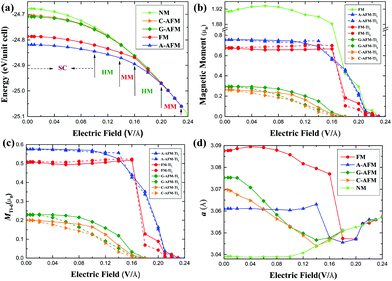Fig. 2 The tuning of the electric field for the NM, FM, A-AFM, C-AFM and G-AFM states: (a) the total energy per unit cell, (b) the total magnetic moments of Ti atoms, (c) the magnetic moments of the 3d-orbital of Ti atoms and (d) the lattice constants. The light green line in (b) is the total magnetic moment per unit cell for the FM state.

Based on the discussions above, for the monolayer Ti2C, the A-AFM state is the most stable ground state when the E ranges from 0 to 0.23 V Å−1. However, when the E > 0.23 V Å−1, the ground state is the NM state. Therefore, we depict the band structures of monolayer Ti2C under different electric fields in Fig. 3(a and b). For the A-AFM state, we find a series of interesting phenomena: (1) when E > 0.02 V Å−1, the band structures of spinning up and spinning down split and the A-AFM state becomes the FIM state; (2) when E = 0.10 V Å−1, it changes from a SC to a spin half-metal (HM), (3) when E = 0.14 V Å−1, it becomes a magnetic metal (MM); (4) when E = 0.16 V Å−1, it becomes a spin HM again; (5) when E = 0.20 V Å−1, it becomes a MM; (6) eventually, when E= 0.23 V Å−1, the magnetism disappears and it becomes a NM metal (Fig. 3(a)). The band gap of the A-AFM state decreases linearly with the increase of E (Fig. 3(c)). In addition, as for the symmetrical structure of monolayer Ti2C, the opposite direction E can not affect the band structures, just the spin-up and the spin-down electrons are exchanged. In Fig. 3(b), when E = 0.26 V Å−1, the NM state changes from metal to SC and the band gap increases with the increase of E in Fig. 3(c). Therefore, a series of electronic phase transitions indicate that the electric field is very important for controlling the performance of the nano electronic devices.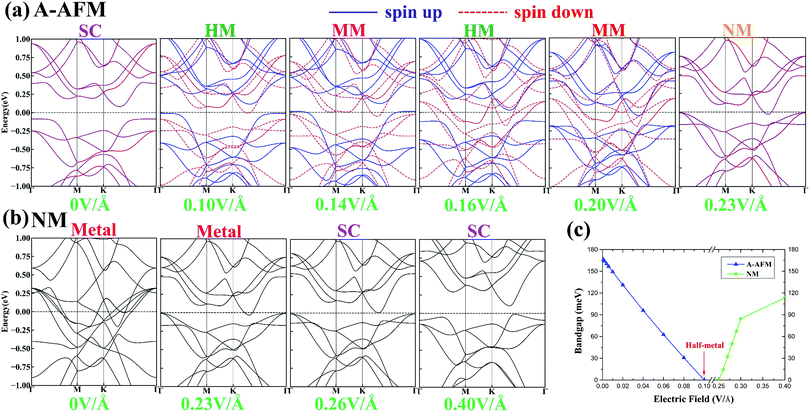Fig. 3 The band structures of A-AFM (a) and NM (b) states under different electric fields. (c) The regulation of band gaps in A-AFM and NM states by electric field.

Because the valence band maximum (VBM) of the A-AFM state is relatively flat in Fig. 3(a), the effective masses (m*) are particularly large. For the effective masses of spin down-VBM (m1*), when E < 0.06 V Å−1, the m1* are too large, so they are not shown in Fig. 4(a). However, when E increases to 0.06 V Å−1, the m1* sharply decrease to 3.1m0 (where m0 is the free electron mass), when E increases to 0.12 V Å−1, m1* decrease to 2.89m0, and when E > 0.12 V Å−1, the spin down-VBM passes through the EF in Fig. 3(a). The effective masses of the spin up and spin down conduction band minimum (CBM) are relatively smaller, 0.64m0, and they have little change with the increase of E. When E increases to 0.08 V Å−1, the effective masses of the spin down-CBM (m2*) decrease to 0.48m0 and the spin down-CBM passes through the EF when E > 0.08 V Å−1. The effective masses of the spin up-CBM (m3*) increase to 1.18m0 at 0.14 V Å−1 and decrease to 0.56m0 at 0.18 V Å−1. When E > 0.23 V Å−1, the magnetic configuration of monolayer Ti2C is the NM state, and when E > 0.26 V Å−1, the NM state is a semiconductor. Therefore, we also calculated m* of the NM state in Fig. 4(b). When E = 0.26 V Å−1, the m* of VBM (m4*) and CBM (m5*) are 2.59m0 and 0.29m0, respectively. The m4* increase to 2.64m0 at 0.3 V Å−1 and decrease to 0.46m0 at 0.4 V Å−1. The m5* change little with the increase of electric field. In summary, m1*, m2*, m3*, and m4* all significantly reduce, which indicates that the carrier mobility and conductivity will increase greatly.38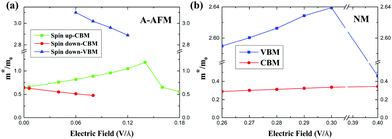Fig. 4 The variation of effective masses in A-AFM (a) and NM (b) states with the electric field.

The MAE is an important parameter in nanoscale magnetic materials.39 By calculating the MAE, the magnetic easy direction can be found, which is important for magnetic materials. The total energies along the high symmetry directions: in-plane (100), (010) and (110); out-of-plane (001) and canted out-of-plane (111) are calculated. The (001) direction has the lowest total energy, so it is the magnetic easy axis and its energy is chosen as a reference. The total energies of the three in-plane directions are the same and the highest, and the MAE is 6.83 μeV per atom. The MAE along the (111) direction is 4.55 μeV per atom. Then, we calculated the MAEs of the xy, xz and yz planes. The MAEs of the xy plane are identical and the MAEs of the xz and yz plane are equal, so, we just show the MAEs of the yz plane in Fig. 5(a). The spin angles θ from 0° to 360° were sampled every 9°. For the monolayer Ti2C, the minimum MAE is at θ = 0° or 180° and the maximum MAE is at θ = 90° or 270°. In Fig. 5(a), the MAEs are strongly dependent on θ, approximating to a sinusoidal relation. Since the monolayer Ti2C is a hexagonal symmetric crystal, the relationship between MAE and θ can be fit by the following formula:40

 MAE(θ) = K1sin2θ + K2sin4θ (1)
where K1 and K2 are magnetic anisotropy constants and θ is the azimuthal angle of rotation. If K1 > 0 and K1 > −K2, the out-of-plane direction (θ = 0°) is the magnetic easy axis; if K1 > 0 and K1 < −K2 or K2 < 0 and K1 < −2K2, the in-planes (θ = 90°) are the magnetic easy directions. According to eqn (1), the fitting values of K1 and K2 are 6.8585 μeV and −0.0417 μeV, which suggests that the z axis is the magnetic easy axis. The three-dimensional MAEs are obtained by rotating the spin axis (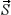) in the whole space in Fig. 5(b and c), which clearly show the MAEs along different directions. The total energy of the (001) direction is always the lowest and the total energy of the (100) direction is the highest when applying an electric field. So, the z axis is still the magnetic easy direction when applying an electric field. Thus, we define MAE = E100E001 (μeV per atom), where E100 and E001 represent the energy per atom of the (100) and (001) directions. As shown in Fig. 5(d), the MAE decreases with the increase of the E; therefore, the results of Fig. 2(b, c) and 5(d) indicate that E can effectively change the spin directions of electrons, causing the directions of spin to be in disorder.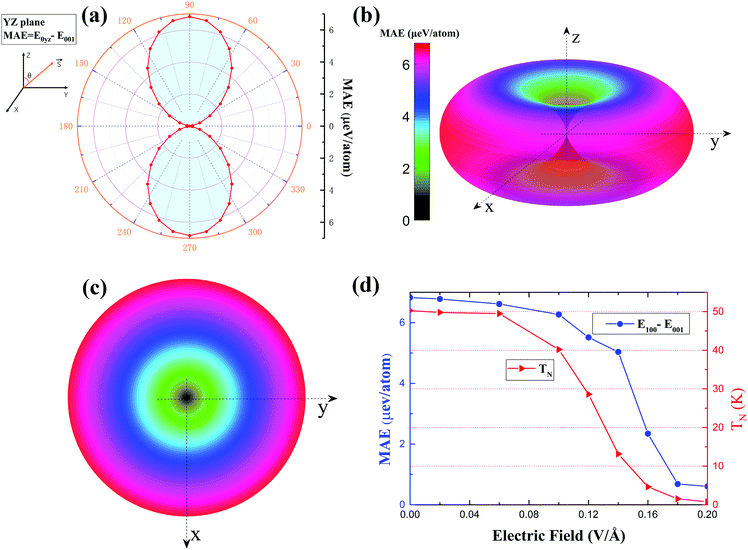Fig. 5 (a) The MAE of different angles θ (degree) in the yz plane, the polar angle 0° is along the (001) direction andis the direction of spin. (b) and (c) are the side and top views of MAE for the whole space, respectively. (d) The MAE and TN vary with the electric field.

In order to determine the exchange coupling of the A-AFM state, the following Heisenberg spin Hamiltonian is used:41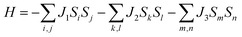(2)
where the parameters of J1, J2 and J3 are the nearest-neighbor (NN), next-nearest-neighbor (NNN) and third-nearest-neighbor (3NN) exchange interactions, as indicated in Fig. 1(a and b). Si is the net spin at the Ti sites i, and (i, j), (k, l) and (m, n) are the Ti atoms of NN, NNN and 3NN, respectively. The energies of DFT are substituted to the Heisenberg spin Hamiltonian, as follows:
 EFM/A-AFM = E0 − (±3J1 + 6J2 ± 3J3)||2 (3)
 EC-AFM/G-AFM = E0 − (±J1 − 2J2 ∓ 3J3)||2 (4)
where EFM/A-AFM is the total energy of the FM/A-AFM state and EC-AFM/G-AFM is the total energy of the C-AFM/G-AFM state. According to Hund's rule, S = 1. J1, J2 and J3 are calculated to be −2.35 meV, 5.95 meV and −0.35 meV, respectively. Because J2 > |J1| ≫ |J3|, the interaction of intralayer Ti atoms are stronger than that of interlayer Ti atoms. J1 < 0 indicates that monolayer Ti2C prefers the AFM state. TN can be estimated from the mean-field approximation formula:42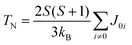(5)
TN of monolayer Ti2C is estimated to be 50 K. According to Fig. 2(a), the energy difference between FM, A-AFM, C-AFM and G-AFM decreases with the increase of the E; so, the values of J also decrease as shown in Table S1 (see the ESI), which leads to the decrease of TN as shown in Fig. 5(d). This indicates that the electric field can effectively reduce TN.

## Conclusions

In this work, we construct 5 magnetic configurations of monolayer Ti2C: NM, FM, A-AFM, C-AFM and G-AFM states. Both the PBE and PBE+U methods show that the A-AFM state has the lowest total energy and its magnetic moment of each Ti atom is the largest, which is 0.757 μB in the PBE method. We predict that monolayer Ti2C is an A-AFM semiconductor material. By applying E, the A-AFM state still has the lowest energy. When E > 0.02 V Å−1, monolayer Ti2C changes from the A-AFM to the FIM state, and then a series of electronic phase transitions occurs: SC (0 V Å−1) ↔ HM (0.1 V Å−1) ↔ MM (0.14 V Å−1) ↔ HM (0.16 V Å−1) ↔ MM (0.2 V Å−1) ↔ NM (0.23 V Å−1) ↔ SC (0.26 V Å−1). With the increase of electric field, the effective masses of A-AFM and NM states both decrease and the conductivity increased significantly. Therefore, the electric field can effectively regulate the conductive properties of monolayer Ti2C. The band gap linearly decreases as E increases from 0 to 0.1 V Å−1, and then increases when E > 0.26 V Å−1. The MAE of monolayer Ti2C is 6.83 μeV per atom and the z axis is the magnetic easy axis while the in-plane MAEs are equal. The MAE decreases and the spin directions of the electrons prefer to disorder as E increases. The mean-field approximation is used to estimate TN = 50 K. TN reduces as the E increases, which provides a good theoretical guidance for reducing the high TN. The regulation of the electronic structures, conductivity and magnetic properties of monolayer Ti2C by an electric field provides exciting prospects for the application of nano magnetic electronic devices.

## Conflicts of interest

There are no conflicts to declare.

## Acknowledgements

We acknowledge the support from the Fundamental Research Funds for the Central Universities (WUT: 2017IB013, 2018IB009 and 2018IB011).

## References

1. K. S. Novoselov, A. K. Geim, S. V. Morozov, D. Jiang, Y. Zhang, S. V. Dubonos, I. V. Grigorieva and A. A. Firsov, Science, 2004, 306, 666–669 CrossRef CAS PubMed.
2. M. Chhowalla, Z. Liu and H. Zhang, Chem. Soc. Rev., 2015, 44, 2584–2586 RSC.
3. D. Pacilé, J. C. Meyer, Ç. Ö. Girit and A. Zettl, Appl. Phys. Lett., 2008, 92, 133107 CrossRef.
4. J. Meyer, S. Hamwi, M. Kroger, W. Kowalsky, T. Riedl and A. Kahn, Adv. Mater., 2012, 24, 5408–5427 CrossRef CAS PubMed.
5. A. Carvalho, M. Wang, X. Zhu, A. S. Rodin, H. Su and A.H. Castro Neto, Nat. Rev. Mater., 2016, 1, 16061 CrossRef CAS.
6. H. Yuan, X. Liu, F. Afshinmanesh, W. Li, G. Xu, J. Sun, B. Lian, A. G. Curto, G. Ye, Y. Hikita, Z. Shen, S. Zhang, X. Chen, M. Brongersma, H. Y. Hwang and Y. Cui, Nat. Nanotechnol., 2015, 10, 707–713 CrossRef CAS PubMed.
7. A. J. Mannix, X. F. Zhou, B. Kiraly, J. D. Wood, D. Alducin, B. D. Myers, X. Liu, B. L. Fisher, U. Santiago, J. R. Guest, M. J. Yacaman, A. Ponce, A. R. Oganov, M. C. Hersam and N. P. Guisinger, Science, 2015, 350, 1513–1516 CrossRef CAS PubMed.
8. M. Naguib, M. Kurtoglu, V. Presser, J. N. Jun Lu, M. Heon, L. Hultman, Y. Gogotsi and M. W. Barsoum, Adv. Mater., 2011, 37, 4248–4253 CrossRef PubMed.
9. M. Naguib, O. Mashtalir, J. Carle, V. Presser, J. Lu, L. Hultman, Y. Gogotsi and M. W. Barsoum, ACS Nano, 2012, 6, 1322–1331 CrossRef CAS PubMed.
10. M. Khazaei, A. Mishra, N. S. Venkataramanan, A. K. Singh and S. Yunoki, Curr. Opin. Solid State Mater. Sci., 2019, 23, 164–178 CrossRef CAS.
11. B. Soundiraraju and B. K. George, ACS Nano, 2017, 11, 8892–8900 CrossRef CAS PubMed.
12. P. Urbankowski, B. Anasori, T. Makaryan, D. Er, S. Kota, P. L. Walsh, M. Zhao, V. B. Shenoy, M. W. Barsoum and Y. Gogotsi, Nanoscale, 2016, 8, 11385–11391 RSC.
13. M. Naguib, J. Halim, J. Lu, K. M. Cook, L. Hultman, Y. Gogotsi and M. W. Barsoum, J. Am. Chem. Soc., 2013, 135, 15966–15969 CrossRef CAS PubMed.
14. M. H. Tran, T. Schäfer, A. Shahraei, M. Dürrschnabel, L. Molina-Luna, U. I. Kramm and C. S. Birkel, ACS Appl. Energy Mater., 2018, 1, 3908–3914 CrossRef CAS.
15. M. Ghidiu, M. Naguib, C. Shi, O. Mashtalir, L. M. Pan, B. Zhang, J. Yang, Y. Gogotsi, S. J. L. Billinge and M. W. Barsoum, Chem. Commun., 2014, 50, 9517–9520 RSC.
16. R. Meshkian, L. Näslund, J. Halim, J. Lu, M. W. Barsoum and J. Rosen, Scr. Mater., 2015, 108, 147–150 CrossRef CAS.
17. J. Zhou, X. Zha, F. Y. Chen, Q. Ye, P. Eklund, S. Du and Q. Huang, Angew. Chem., Int. Ed., 2016, 55, 5008–5013 CrossRef CAS PubMed.
18. Y. Yang, S. Umrao, S. Lai and S. Lee, J. Phys. Chem. Lett., 2017, 8, 859–865 CrossRef CAS PubMed.
19. B. Xu, M. Zhu, W. Zhang, X. Zhen, Z. Pei, Q. Xue, C. Zhi and P. Shi, Adv. Mater., 2016, 28, 3333–3339 CrossRef CAS PubMed.
20. N. C. Frey, A. Bandyopadhyay, H. Kumar, B. Anasori, Y. Gogotsi and V. B. Shenoy, ACS Nano, 2019, 13, 2831–2839 CrossRef CAS PubMed.
21. X. Zha, Q. Huang, J. He, H. He, J. Zhai, J. S. Francisco and S. Du, Sci. Rep., 2016, 6, 27971 CrossRef PubMed.
22. Y. Bai, K. Zhou, N. Srikanth, J. H. L. Pang, X. He and R. Wang, RSC Adv., 2016, 6, 35731–35739 RSC.
23. X. Yu, Y. Li, J. Cheng, Z. Liu, Q. Li, W. Li, X. Yang and B. Xiao, ACS Appl. Mater. Interfaces, 2015, 7, 13707–13713 CrossRef CAS PubMed.
24. Y. Xie and P. R. C. Kent, Phys. Rev. B: Condens. Matter Mater. Phys., 2013, 87, 235441 CrossRef.
25. P. Chakraborty, T. Das, D. Nafday, L. Boeri and T. Saha-Dasgupta, Phys. Rev. B, 2017, 95, 184106 CrossRef.
26. Q. Wan, S. Li and J. Liu, ACS Appl. Mater. Interfaces, 2018, 10, 6369–6377 CrossRef CAS PubMed.
27. C. Ke, Y. Wu, G. Guo, W. Lin, Z. Wu, C. Zhou and J. Kang, Phys. Rev. Appl., 2018, 9, 44029 CrossRef CAS.
28. Y. Zhou, Z. Peng, Y. Chen, K. Luo, J. Zhang and S. Du, Comput. Mater. Sci., 2019, 168, 137–143 CrossRef CAS.
29. G. Kresse and J. Furthmüller, Phys. Rev. B: Condens. Matter Mater. Phys., 1996, 54, 11169–11186 CrossRef CAS PubMed.
30. J. P. Perdew, K. Burke and M. Ernzerhof, Phys. Rev. Lett., 1996, 77, 3865–3868 CrossRef CAS PubMed.
31. G. Kresse and D. Joubert, Phys. Rev. B: Condens. Matter Mater. Phys., 1999, 59, 1758–1775 CrossRef CAS.
32. M. Sternik and U. D. Wdowik, Phys. Chem. Chem. Phys., 2018, 20, 7754–7763 RSC.
33. S. Wang, J. Li, Y. Du and C. Cui, Comput. Mater. Sci., 2014, 83, 290–293 CrossRef CAS.
34. Z. Guo, J. Zhou, C. Si and Z. Sun, Phys. Chem. Chem. Phys., 2015, 17, 15348–15354 RSC.
35. I. R. Shein and A. L. Ivanovskii, Comput. Mater. Sci., 2012, 65, 104–114 CrossRef CAS.
36. L. Gan, Y. Zhao, D. Huang and U. Schwingenschlögl, Phys. Rev. B: Condens. Matter Mater. Phys., 2013, 87, 245307 CrossRef.
37. G. Gao, G. Ding, J. Li, K. Yao, M. Wu and M. Qian, Nanoscale, 2016, 8, 8986–8994 RSC.
38. S. Qian, X. Sheng, X. Xu, Y. Wu, N. Lu, Z. Qin, J. Wang, C. Zhang, E. Feng, W. Huang and Y. Zhou, J. Mater. Chem. C, 2019, 7, 3569–3575 RSC.
39. H. Y. Lv, W. J. Lu, X. Luo, X. B. Zhu and Y. P. Sun, Phys. Rev. B, 2019, 99, 134416 CrossRef CAS.
40. B. Wang, Y. Zhang, L. Ma, Q. Wu, Y. Guo, X. Zhang and J. Wang, Nanoscale, 2019, 11, 4204–4209 RSC.
41. W. B. Zhang, Q. Qu, P. Zhu and C. H. Lam, J. Mater. Chem. C, 2015, 3, 12457–12468 RSC.
42. W. Sun, Y. Xie and P. R. Kent, Nanoscale, 2018, 10, 11962–11968 RSC.
43. Y.-X. Yu, J. Phys. Chem. C, 2019, 123, 205–213 CrossRef CAS.
44. Y.-X. Yu, J. Phys. Chem. C, 2016, 120, 5288–5296 CrossRef CAS.
45. Y. Xin and Y.-X. Yu, Mater. Des., 2017, 130, 512–520 CrossRef CAS.
46. F. Zhang, W. Mi and X. Wang, Adv. Electron. Mater., 2020, 6, 1900778 CrossRef CAS.
47. X. Ma and W. Mi, J. Phys. Chem. C, 2020, 124, 3095–3106 CrossRef CAS.
48. X. Ma, L. Yin, J. Zou, W. Mi and X. Wang, J. Phys. Chem. C, 2019, 123, 17440–17448 CrossRef CAS.
49. F. Zhang, W. Mi and X. Wang, Nanoscale, 2019, 11, 10329–10338 RSC.
50. R. Gholizadeh and Y.-X. Yu, J. Phys. Chem. C, 2014, 118, 28274–28282 CrossRef CAS.

### Footnote

Electronic supplementary information (ESI) available. See DOI: 10.1039/d0cp00507j
 This journal is © the Owner Societies 2020